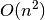Warning

This documents an unmaintained version of NetworkX. Please upgrade to a maintained version and see the current NetworkX documentation.

# random_geometric_graph¶

random_geometric_graph(n, radius, dim=2, pos=None)[source]

Returns a random geometric graph in the unit cube.

The random geometric graph model places n nodes uniformly at random in the unit cube. Two nodes are joined by an edge if the Euclidean distance between the nodes is at most radius.

Parameters: n (int) – Number of nodes radius (float) – Distance threshold value dim (int, optional) – Dimension of graph pos (dict, optional) – A dictionary keyed by node with node positions as values. Graph

Examples

Create a random geometric graph on twenty nodes where nodes are joined by an edge if their distance is at most 0.1:

>>> G = nx.random_geometric_graph(20, 0.1)


Notes

This algorithm currently only supports Euclidean distance.

This uses analgorithm to build the graph. A faster algorithm is possible using k-d trees.

The pos keyword argument can be used to specify node positions so you can create an arbitrary distribution and domain for positions.

For example, to use a 2D Gaussian distribution of node positions with mean (0, 0) and standard deviation 2:

>>> import random
>>> n = 20
>>> p = {i: (random.gauss(0, 2), random.gauss(0, 2)) for i in range(n)}
>>> G = nx.random_geometric_graph(n, 0.2, pos=p)


References

  Penrose, Mathew, Random Geometric Graphs, Oxford Studies in Probability, 5, 2003.